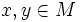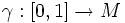# Riemannian manifold is metric space

(diff) ← Older revision | Latest revision (diff) | Newer revision → (diff)

## Statement

Any path-connected Riemannian manifold naturally acquires the structure of a metric space.

## Explanation

Suppose$M$ is a path-connected differential manifold and$g$ is a Riemannian metric on$M$. For two points$x,y \in M$, let$\gamma:[0,1] \to M$ be a path from$x$ to$y$ and define the length of$\gamma$ as:$l(\gamma) = \int_0^1 |\gamma'(t)| dt$

where the modulus sign is the norm in the tangent space with respect to the Riemannian metric. The distance between$x[itex] and [itex]y$ is defined as the infimum of the lengths of all paths from$x$ to$y$.

## Facts

### Geodesic completeness

A complete Riemannian manifold, or geodesically complete Riemannian manifold, is one where at any point, the exponential map from the tangent space at the point to the manifold, is well-defined. Complete Riemannian manifolds give rise to complete metric spaces by the Hopf-Rinow theorem. The metric spaces they give rise to are also geodesic metric spaces. Not every geodesic metric space arises from a complete Riemannian manifold (in particular, for instance, the open disc is far from complete but it is a geodesic metric space).

### Submanifolds

Given a Riemannian manifold and a Riemannian submanifold the metric space structure arising from the Riemannian metric on the submanifold, is different from the metric space structure arising from the Riemannian metric on the manifold, inherited to the submanifold.